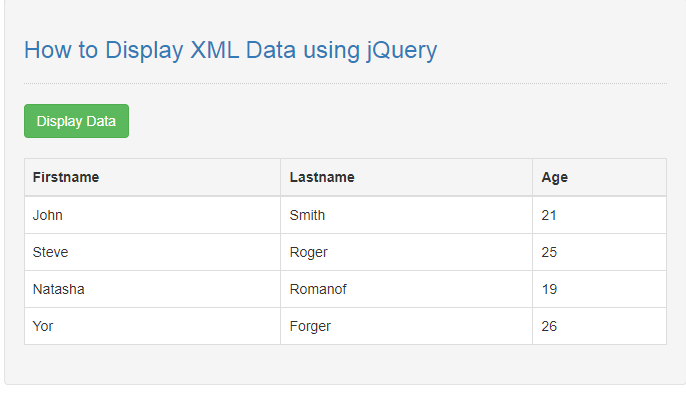# How to Display XML Data using jQuery

## Introduction

In this tutorial we will create a How to Display XML Data using jQuery. This tutorial purpose is to teach how to you will display the xml data into the html page. This will tackle the process for display all the xml data. I will provide a sample program to show the actual coding of this tutorial.

This tutorial is very easy to understand just follow the instruction I provided and you can do it without a problem. This program can be use if you want to secure some important data in your site. I will try my best to give you the easiest way of creating this program Displaying of xml. So let's do the coding.

## Before we get started:

Here is the link for the jQuery that i used in this tutorial https://jquery.com/.

Lastly, this is the link for the template that i used for the layout design https://getbootstrap.com/.

## Creating The Interface

This is where we will create a simple interface for our application. This code will display only an empty table and a simple button. To create this simply copy and write it into your text editor, then save it as index.html.
`<!DOCTYPE html><html lang="en">	<head>		<meta charset="UTF-8" name="viewport" content="width=device-width, initial-scale=1"/>		<link rel="stylesheet" type="text/css" href="css/bootstrap.css"/>	</head><body>	<nav class="navbar navbar-default">		<div class="container-fluid">			<a class="navbar-brand" href="https://sourcecodester.com">Sourcecodester</a>		</div>	</nav>	<div class="col-md-3"></div>	<div class="col-md-6 well">		<h3 class="text-primary">How to Display XML Data using jQuery</h3>		<hr style="border-top:1px dotted #ccc;"/>		<button type="button" class="btn btn-success" id="display">Display Data</button>		<br /><br />		<table class="table table-bordered">			<thead>				<tr>					<th>Firstname</th>					<th>Lastname</th>					<th>Age</th>				</tr>			</thead>			<tbody id="result" style="background-color:#fff;"></tbody>		</table>	</div></body><script src="js/jquery-3.2.1.min.js"></script><script src="script.js"></script></html>`

## Creating JavaScript Function

This is where the main function of the application is. This code will automatically translate and display the xml data into the html table. To do this just copy and write these block of codes inside the text editor and save it as script.js.
`\$(document).ready(function(){ 	let xml = 	"<?xml version='1.0' ?>"	+ "<doc>"	+"		<member>"	+"			<firstname>John</firstname>"	+"			<lastname>Smith</lastname>"	+"			<age>21</age>"	+"		</member>"	+"		<member>"	+"			<firstname>Steve</firstname>"	+"			<lastname>Roger</lastname>"	+"			<age>25</age>"	+"		</member>"	+"		<member>"	+"			<firstname>Natasha</firstname>"	+"			<lastname>Romanof</lastname>"	+"			<age>19</age>"	+"		</member>"	+"		<member>"	+"			<firstname>Yor</firstname>"	+"			<lastname>Forger</lastname>"	+"			<age>26</age>"	+"		</member>"	+"		<member>"	+"			<firstname>Tony</firstname>"	+"			<lastname>Stark</lastname>"	+"			<age>35</age>"	+"		</member>"	+"	</doc>";  	\$('#display').on('click', function(){		let data = \$.parseXML(xml);  		let \$xml = \$(data); 		let \$member = \$xml.find("member"); 		let content = "";		\$member.each(function(){ 			let firstname = \$(this).find('firstname').text();			let lastname = \$(this).find('lastname').text();			let age = \$(this).find('age').text(); 			content += "<tr>"				+ "<td>"+firstname+"</td>"				+ "<td>"+lastname+"</td>"				+ "<td>"+age+"</td>"				+ "</tr>"; 			\$("#result" ).html(content); 		});	});  });`

In the given code we first call the basic jQuery ready event to signal that the DOM of the page is now ready to be use. After that we create new variable that will be the xml data and called it xml.We then bind the button trigger event using the on() function. We first then set a variable called data that will parse the our xml data using the \$.parseXML() function and set it to a new variable called \$xml. Next we will need to find a specific data using the find() function and set it as \$member variable.

In order to display the xml data into the table we need to loop the data by calling the parent data first and finding the children data, then append all of it using the html() function

## Output: